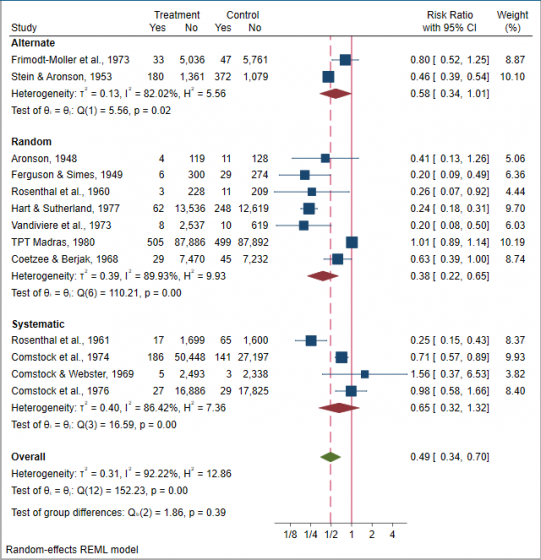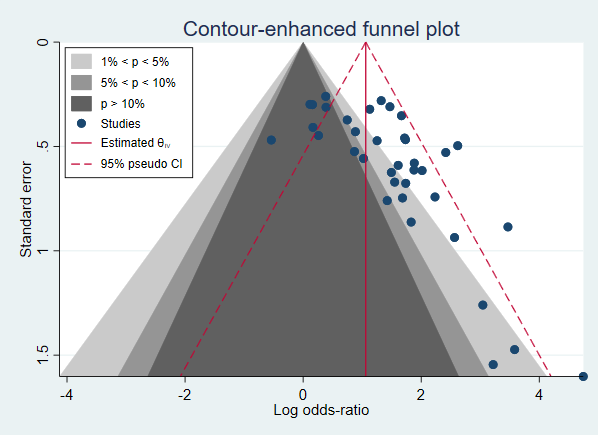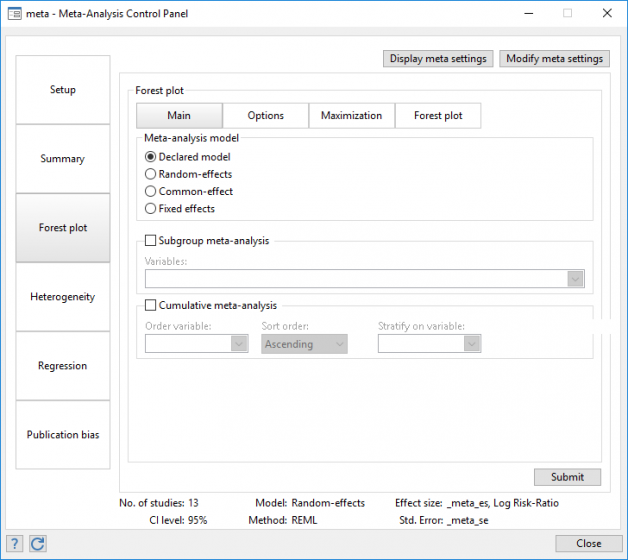Watch Meta-analysis in Stata.

Data setup and effect sizes

Effect sizes for binary data

Odds ratio

Peto’s odds ratio

Risk ratio

Risk difference

Effect sizes for continuous data

Hedges’s g

Cohen’s d

Glass’s delta (two versions)

Unstandardized mean difference

Generic (precomputed) effect sizes

Different methods for zero-cells adjustment with binary data

Update declared meta-analysis settings at any time

Describe declared meta-analysis settings

Meta-analysis models

Common-effect model

Inverse-variance method

Mantel–Haenszel method

Fixed-effects model

Inverse-variance method

Mantel–Haenszel method

Random-effects model

Iterative methods: REML, MLE, and empirical Bayes

Noniterative methods: DerSimonian–Laird, Hedges, Sidik–Jonkman, and Hunter–Schmidt

Prediction intervals

Sensitivity analysis: User-specified values for heterogeneity parameters tau2 and I2

Meta-analysis summary

Standard meta-analysis

Forest plots

Subgroup meta-analysis

One grouping variable

Multiple grouping variables

Subgroup forest plots

Cumulative meta-analysis

Standard analysis

Stratified analysis

Cumulative forest plots

Forest plots

Standard forest plot

Custom forest plot

Subgroup forest plot

Cumulative forest plot

Cropped CI rangesHeterogeneity

Basic summary

Forest plots

L’Abbé plots for binary data

Subgroup meta-analysis

Meta-regression

Bubble plots

Meta-regression

Continuous and categorical moderators

Fixed-effects and random-effects regression

Multiplicative and additive residual heterogeneity

Postestimation features

Fitted values

Residuals

Random effects

Standard errors of predicted quantities

Bubble plots

Other standard postestimation tools such as marginscontrasts, and more

Small-study effects

Funnel plots

Tests for small-study effects

Funnel plots

Standard funnel plots

Contour-enhanced funnel plots

Two-sided or one-sided significance contours

Multiple precision metrics for the y-axis

Tests for funnel-plot asymmetry or small-study effects

Egger regression-based test

Harbord regression-based test

Peters regression-based test

Begg rank correlation test

Adjust for moderators to account for heterogeneity

Traditional and random-effects versions

Stratified funnel plots

Fully customizable

Publication bias

Funnel plots

Tests for funnel-plot assymetry

Nonparametric trim-and-fill method

Three estimators for number of missing studies

Impute studies on the left or right side of the funnel plot

Nine estimation methods for the iteration stage

Nine estimation methods for the pooling stage

Choose the side of the funnel plot with missing studies

Standard and contour-enhanced funnel plot for the observed and imputed studies.Control panel

Set up data and compute effect sizes

Update specific characteristics at any time

Summarize results in tables and produce forest plots

Perform subgroup analysis and cumulative meta-analysis

Perform meta-regression and pick from a variety of postestimation tools

Perform publication bias analysis